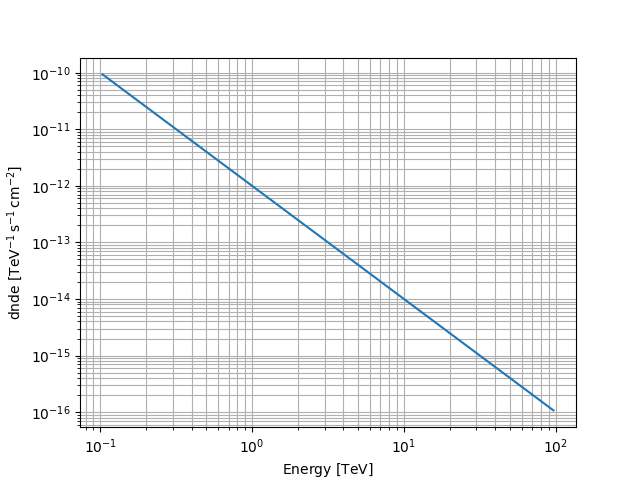# Power law spectral model#

This model parametrises a power law spectrum.

It is defined by the following equation:

$\phi(E) = \phi_0 \cdot \left( \frac{E}{E_0} \right)^{-\Gamma}$

## Example plot#

Here is an example plot of the model:

from astropy import units as u
import matplotlib.pyplot as plt
from gammapy.modeling.models import Models, PowerLawSpectralModel, SkyModel

energy_bounds = [0.1, 100] * u.TeV
model = PowerLawSpectralModel(
index=2,
amplitude="1e-12 TeV-1 cm-2 s-1",
reference=1 * u.TeV,
)
model.plot(energy_bounds)
plt.grid(which="both")## YAML representation#

Here is an example YAML file using the model:

model = SkyModel(spectral_model=model, name="power-law-model")
models = Models([model])

print(models.to_yaml())

components:
-   name: power-law-model
type: SkyModel
spectral:
type: PowerLawSpectralModel
parameters:
-   name: index
value: 2.0
-   name: amplitude
value: 1.0e-12
unit: cm-2 s-1 TeV-1
-   name: reference
value: 1.0
unit: TeV


Gallery generated by Sphinx-Gallery